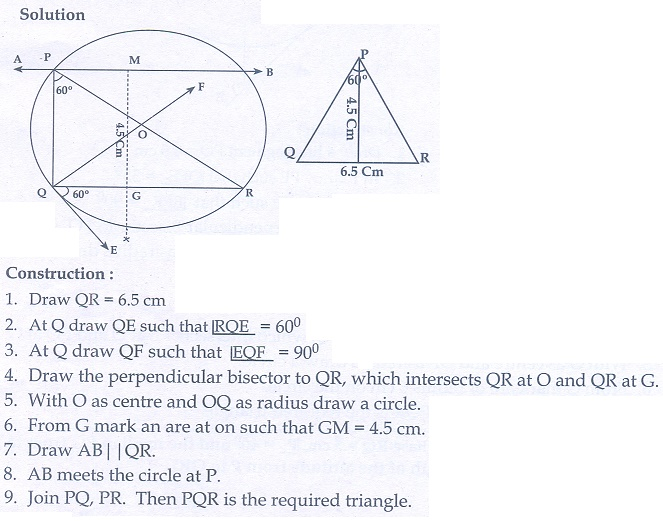Home | | Maths 10th Std | Exercise 4.2: Thales Theorem and Angle Bisector Theorem

# Exercise 4.2: Thales Theorem and Angle Bisector Theorem

Construction of triangle using Theorem 1: Basic Proportionality Theorem (BPT) or Thales theorem, Theorem 2: Converse of Basic Proportionality Theorem, Theorem 3: Angle Bisector Theorem, Theorem 4: Converse of Angle Bisector Theorem (Maths Book back answers and solution for Exercise questions)

Exercise 4.2

1. In ΔABC , D and E are points on the sides AB and AC respectively such that DE|| BC

(i) If AD/DB = 3/4 and AC = 15 cm find AE

(ii). If AD = 8x − 7 , DB = 5x − 3 , AE = 4x − 3 and EC = 3x −1 , find the value of x.2. ABCD is a trapezium in which AB || DC and P,Q are points on AD and BC respectively, such that PQ || DC if PD = 18 cm, BQ = 35 cm and QC = 15 cm, find AD.3. In ΔABC, D and E are points on the sides AB and AC respectively. For each of the following cases show that DE || BC

(i) AB = 12 cm, AD = 8 cm, AE = 12 cm and AC = 18 cm.

(ii) AB = 5.6 cm, AD = 1.4 cm, AC = 7.2 cm and AE = 1.8 cm.4. In fig. if PQ || BC and PR || CD prove that5. Rhombus PQRB is inscribed in ΔABC such that B is one of its angle. PQ and R lie on ABAC and BC respectively. If AB= 12 cm and BC = 6 cm, find the sides PQ, RB of the rhombus.6. In trapezium ABCDAB || DC , E and F are points on non-parallel sides AD and BC respectively, such that EF || AB . Show that.7. In figure DE || BC and CD || EF . Prove that AD AB ×AF .8. In ΔABC,  AD is the bisector of A meeting side BC at D, if AB 10 cm, AC = 14 cm and BC = 6 cm, find BD and DC9. Check whether AD is bisector of A of ΔABC in each of the following

(i) AB = 5 cm, AC = 10 cm, BD=1.5 cm and CD= 3.5 cm.

(ii) AB= 4 cm, AC = 6 cm, BD = 1.6 cm and CD= 2.4 cm.10. In figure QPR = 90° , PS is its bisector. If ST ┴ PR , prove that ST×(PQ+PR)=PQ×PR.11. ABCD is a quadrilateral in which AB=AD, the bisector of BAC and CAD intersect the sides BC and CD at the points E and F respectively. Prove that EF || BD .12. Construct a ΔPQR which the base PQ = 4.5 cm, R = 35 and the median from R to RG is 6 cm.13. Construct a ΔPQR in which QR = 5 cm, P = 40° and the median PG from P to QR is 4.4 cm. Find the length of the altitude from P to QR.14. Construct a ΔPQR such that QR = 6.5 cm, P = 60° and the altitude from P to QR is of length 4.5 cm.15. Construct a ΔABC such that AB = 5.5 cm, C = 25° and the altitude from C to AB is 4 cm.16. Draw a triangle ABC of base BC = 5.6 cm, A = 40° and the bisector of A meets BC at D such that CD = 4 cm.17. Draw ΔPQR such that PQ = 6.8 cm, vertical angle is 50° and the bisector of the vertical angle meets the base at D where PD = 5.2 cm.1.(i) 6.43 cm (ii) 1

2. 60 cm

5. 4 cm, 4 cm

8. 2.5 cm, 3.5 cm

9.(i) Not a bisector (ii) Bisector

13. 2.1 cm

Tags : Problem Questions with Answer, Solution | Mathematics , 10th Mathematics : UNIT 4 : Geometry
Study Material, Lecturing Notes, Assignment, Reference, Wiki description explanation, brief detail
10th Mathematics : UNIT 4 : Geometry : Exercise 4.2: Thales Theorem and Angle Bisector Theorem | Problem Questions with Answer, Solution | Mathematics